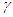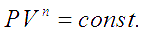# Gas Compression

Gas compression is done to increase the pressure of the gas, this is accompanied by change of state of the gas which means change in temperature and volume of a quantum of gas going under compression. If the pressure of gas is raised from P1 to P2, the compression ratio for this process is defined as, P1 / P2. The physical state of gas will undergo change depending on the process, whether it is isothermal, adiabatic or typically polytropic.

Adiabatic compression: For gas compressors without any cooling, gas temperature rises with rise in pressure. The temperature rise is given by the equation,Here the subscripts 1 and 2 correspond to initial and final states of the gas respectively.is the ratio of specific heat at constant pressure to specific heat for constant volume for a gas (Cp/Cv). For such an adiabatic process, the head developed and power consumed by the compressor are given by following two equations.This developed pressure head when multiplied by the inlet volumetric flowrate of the gas (V) gives shaft power required to drive an ideal adiabatic compressor, given by following equation,In these equations R is the ‘Gas constant’ which is obtained by dividing the ‘Universal gas constant’ by molecular weight of a particular gas.

Isothermal compression: When the gas being compressed in a compressor is cooled with jacketed flow of a coolant, the process is an isothermal process. The work done in this case and hence the power required to run this type of cooled compressors is the theoretically minimum possible limit. This minimum possible power to compress a gas from P1 to P2 is given by following equation,Here subscripts 1 and 2 correspond to inlet and outlet states of the gas, V is the inlet volumetric flow of gas.

Polytropic compression: Adiabatic (constant entropy) and isothermal (constant temperature) compression processes are ideal and theoretical in nature. The actual compression processes are polytropic and typically described with the equation,Note that when n is replaced by, this process becomes adiabatic. If, n >the work done for polytropic compression and power required to run such a compressor is more than that required for and ideal frictionless adiabatic compressor. We get the head developed and power required for polytropic gas compression by replacingby n in the equations for adiabatic gas compression.In these equations R is the ‘Gas constant’ which is obtained by dividing the ‘Universal gas constant’ by molecular weight of a particular gas. V is inlet volumetric flow of gas.